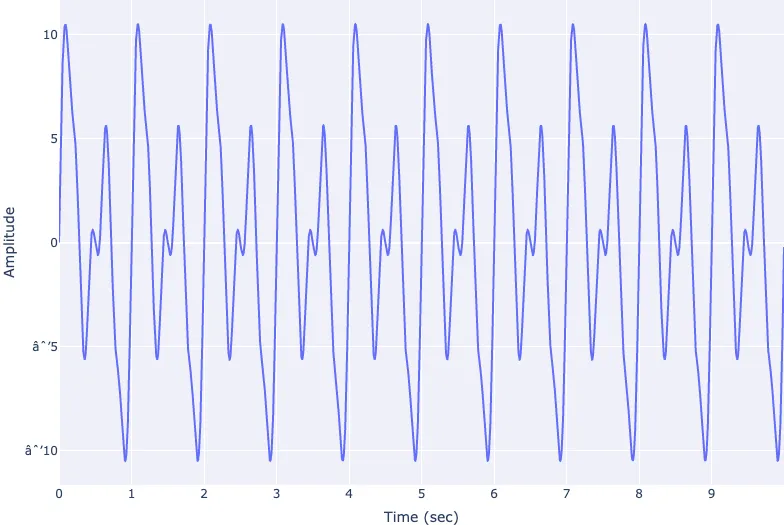## Signal Processing

Signal processing basics and EEG.## Preamble

import numpy as np                   # for multi-dimensional containers
import pandas as pd                  # for DataFrames
import plotly.graph_objects as go    # for data visualisation
from plotly.subplots import make_subplots


## Creating Multiple Sine Wave

Let's create and plot five sine waves, similar to the ones from the previous section. Let's start by defining our time window and sample rate.

sample_rate = 1000
start_time = 0
end_time = 10
theta = 0

time = np.arange(start_time, end_time, 1/sample_rate)


Now we'll store their frequencies in a list named frequency, and their amplitudes in a list named amplitude.

frequency = [3, 5, 2, 1, 10]
amplitude = [1, 2, 7, 3, 0.1]


Finally, let's loop through our five frequency/amplitude values and use them to calculate and visualise the sine waves using subplots.

fig = make_subplots(rows=5, cols=1, shared_xaxes=True)

for i in range(5):
sinewave = amplitude[i] * np.sin(2 * np.pi * frequency[i] * time + theta)
fig.add_scatter(x=time, y=sinewave, row=i+1, col=1, name=f"wave {i+1}")

fig.show()